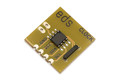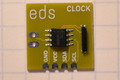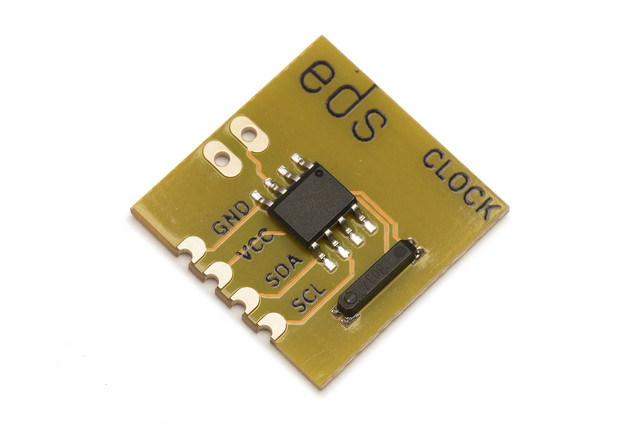# Electric Dollar Store

•••• ## I²C real time clock calendar

• HT1382 Real Time Clock with a 32 kHz crystal
• onboard precision crystal
• calendar valid until 2099
• terminals for external battery backup
• standard 18x18mm module with I²C and power

• \$1.50

CLOCK is a real-time clock and calendar. There is an external connection for a backup battery. The HT1382 automatically switches to the battery supply when the VCC supply is lost. For additional precision, the HT1382 has a clock compensation control, which allows trimming the crystal frequency to within 1ppm.

```import sys
import time

from i2cdriver import I2CDriver, EDS

if __name__ == '__main__':
i2 = I2CDriver(sys.argv)

d = EDS.Clock(i2)
d.set()
while 1:
time.sleep(1)
```
```#include <Wire.h>

class clock {
int a;
public:
int
year;       // 2000 - 2099
byte
month,      // 1 - 12
mday,       // 1 - 31
hour,       // 0 - 24
minute,     // 0 - 60
second,     // 0 - 60
weekday;    // Day of week 1-7

void begin(byte _a = 0x68) {
a = _a;
}
byte bcd(byte x) {
return (x % 10) + 16 * (x / 10);
}
byte decimal(byte x) {
return (x & 0xf) + 10 * (x >> 4);
}
void set() {
Wire.beginTransmission(a);
Wire.write(7);
Wire.write(0);
Wire.endTransmission();

Wire.beginTransmission(a);
Wire.write(0);
Wire.write(bcd(second));
Wire.write(bcd(minute));
Wire.write(0x80 | bcd(hour));
Wire.write(bcd(mday));
Wire.write(bcd(month));
Wire.write(weekday);
Wire.write(bcd(year % 100));
Wire.endTransmission();
}
Wire.beginTransmission(a);
Wire.write(0);
Wire.endTransmission(false);
Wire.requestFrom(a, 7);
}
};

clock Clock;

void setup() {
Serial.begin(115200);
Wire.begin();
Clock.begin();

Clock.year    = 2019;
Clock.month   = 4;
Clock.mday    = 1;
Clock.hour    = 8;
Clock.minute  = 15;
Clock.second  = 0;

Clock.set();
}

void loop() {

Serial.print(" year:");   Serial.print(Clock.year);
Serial.print(" month:");  Serial.print(Clock.month);
Serial.print(" mday:");   Serial.print(Clock.mday);

Serial.print(" hour:");   Serial.print(Clock.hour);
Serial.print(" minute:"); Serial.print(Clock.minute);
Serial.print(" second:"); Serial.print(Clock.second);

Serial.println();
delay(1000);
}
```
```from machine import I2C
import struct
import time
import utime

class Clock:
""" CLOCK is a HT1382 I2C/3-Wire Real Time Clock with a 32 kHz crystal """
def __init__(self, i2, a = 0x68):
self.i2 = i2
self.a = a

def set(self, tt = None):
""" tt is (year, month, mday, hour, minute, second, weekday, yearday), as used
by utime. """
if tt is None:
tt = utime.localtime()
(year, month, mday, hour, minute, second, weekday, yearday) = tt
def bcd(x):
return (x % 10) + 16 * (x // 10)
self.i2.writeto_mem(self.a, 7, bytes())
self.i2.writeto_mem(self.a, 0, bytes([
bcd(second),
bcd(minute),
0x80 | bcd(hour),    # use 24-hour mode
bcd(mday),
bcd(month),
1 + weekday,
bcd(year % 100)]))

def regrd(self, addr, fmt = "B"):
return struct.unpack(fmt, b)

(ss,mm,hh,dd,MM,ww,yy) = self.regrd(0, "7B")
def dec(x):
return (x % 16) + 10 * (x // 16)
return (
2000 + dec(yy),
dec(MM),
dec(dd),
dec(hh & 0x7f),
dec(mm),
dec(ss),
dec(ww) - 1)

def main():
i2 = I2C(1, freq = 100000)

d = Clock(i2)

# Set the clock to 2010-2-10 14:45:00
d.set((2019, 2, 10, 14, 45, 0, 0, 1))

while True:
print('year=%4d month=%2d mday=%2d time=%02d:%02d:%02d weekday=%d' % d.read())
time.sleep(1)
```

 Default I²C address `0x68` (`0b1101000`) Current consumption (typ.) 0.1 mA Vcc 2.8 - 5.5 V